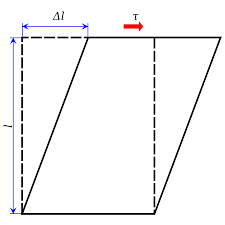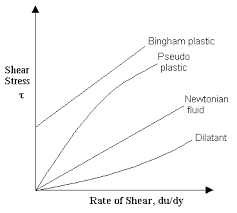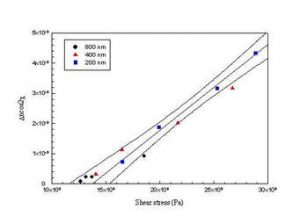## How to Calculate and Solve for Shear Stress | Material SelectionThe image above represents shear stress.

To compute for shear stress, three essential parameters are needed and these parameters are Twisting Moment (M), Radius (r) and Polar Moment of Inertia (J).

The formula for calculating shear stress:

τ = Mr/J

Where:

τ = Shear Stress
M = Twisting Moment
J = Polar Moment of Inertia

Let’s solve an example;
Find the shear stress when the twisting moment is 12, the radius is 8 and the polar moment of inertia is 14.

This implies that;

M = Twisting Moment = 12
J = Polar Moment of Inertia = 14

τ = Mr/J
τ = (12)(8)/14
τ = 96/14
τ = 6.85

Therefore, the shear stress is 6.85 Pa.

Calculating the Twisting Moment when the Shear Stress, the Radius and the Polar Moment of Inertia is Given.

M = τJ / r

Where;

M = Twisting Moment
τ = Shear Stress
J = Polar Moment of Inertia

Let’s solve an example;
Find the twisting moment when the shear stress is 10, the radius is 6 and the polar moment of inertia is 4.

This implies that;

τ = Shear Stress = 10
J = Polar Moment of Inertia = 4

M = τJ / r
M = 10 x 4 / 6
M = 40 / 6
M = 6.67

Therefore, the twisting moment is 6.67.

## How to Calculate and Solve for Viscosity of Newtonian Fluids | RheologyThe image above represents viscosity of newtonian fluids.

To compute for viscosity of newtonian fluids, two essential parameters are needed and these parameters are Shear Stress (ζ) and Shear Rate (γ).

The formula for calculating viscosity of newtonian fluids:

η = ζ / γ

Where:

η = Viscosity of Newtonian Fluids
ζ = Shear Stress
γ = Shear Rate

Let’s solve an example;
Find the viscosity of newtonian fluids when the shear stress is 15 and the shear rate is 5.

This implies that;

ζ = Shear Stress = 15
γ = Shear Rate = 5

η = ζ / γ
η = 15 / 5
η = 3

Therefore, the viscosity of newtonian fluids is 3.

Calculating for Shear Stress when the Newtonian Fluids and the Shear Rate is Given.

ζ = η x γ

Where;

ζ = Shear Stress
η = Viscosity of Newtonian Fluids
γ = Shear Rate

Let’s solve an example;
Find the shear stress when the newtonian fluids is 20 and the shear rate is 4.

This implies that;

η = Viscosity of Newtonian Fluids = 20
γ = Shear Rate = 4

ζ = η x γ
ζ = 20 x 4
ζ = 80

Therefore, the shear stress is 80.

## How to calculate and Solve for Shear Stress | Rock MechanicsThe image above represents shear stress.

To compute for shear stress, two essential parameters are needed and these parameters are Shear Force (ΔS) and Area (ΔA).

The formula for calculating the shear stress:

τ = ΔS / ΔA

Where:

τ = Shear Stress
ΔS = Shear Force
ΔA = Area

Let’s solve an example;
Find the shear stress when the shear force is 16 with an area of 30.

This implies that;

ΔS = Shear Force = 16
ΔA = Area = 30

τ = ΔS / ΔA
τ = 16 / 30
τ = 0.53

Therefore, the shear stress is 0.53 Pa.

Calculating the Shear Force when the Shear Stress and Area is Given.

ΔS = τ x ΔA

Where;

ΔS = Shear Force
τ = Shear Stress
ΔA = Area

Let’s solve an example;
Find the shear force with a shear stress of 40 and an area of 12.

This implies that;

τ = Shear Stress = 40
ΔA = Area = 12

ΔS = τ x ΔA
ΔS = 40  x 12
ΔS = 480

Therefore, the Shear Force is 480.